# Two angles

The triangles ABC and A'B'C 'are similar. In the ABC triangle, the two angles are 25° and 65°. Explain why in the triangle A'B'C 'is the sum of two angles of 90 degrees.

Result

x =  90 °

#### Solution:Leave us a comment of example and its solution (i.e. if it is still somewhat unclear...):

Showing 0 comments:Be the first to comment!#### To solve this example are needed these knowledge from mathematics:

See also our trigonometric triangle calculator.

## Next similar examples:

1. Angles in triangle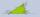Calculate the alpha angle in the triangle if beta is 61 degrees and 98 gamma degrees.
2. Triangle radians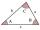The size of two internal angles of a triangle ABC are α=6/18π and β=7/18π. Calculate the size of the third angle.
3. 3-bracket 3Two angles in a triangle are 90° and 60°. Has triangle at least two equal sides?
4. Triangle P2Can triangle have two right angles?
5. SimilarityAre two right triangles similar to each other if the first one has a acute angle 70° and second one has acute angle 20°?
6. Rhombus anglesIf one angle in the rhombus is 159°, what is it neighboring angle in rhombus?
7. Rectangular trapezoid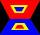How many inner right angles has a rectangular trapezoid?
8. Parallelogram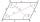In the parallelogram we know one internal angle 67°33`. Calculate the other internal angles.
9. Angles 1It is true neighboring angles have not common arm?
10. 3-bracketMay be the largest angle in the triangle less than 20°?
11. Chord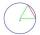Point on the circle is the end point of diameter and end point of chord length of radius. What angle between chord and diameter?
12. DiagonalCan a rhombus have the same length diagonal and side?
13. MidpointsTriangle whose sides are midpoints of sides of triangle ABC has a perimeter 45. How long is perimeter of triangle ABC?
14. QuizQ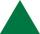An isosceles triangle has two sides of length 7 km and 39 km. How long is a third side?
15. Obtuse angleWhich obtuse angle is creating clocks at 17:00?
16. Simple equationSolve the following simple equation: 2. (4x + 3) = 2-5. (1-x)
17. TeacherTeacher Rem bought 360 pieces of cupcakes for the outreach program of their school. 5/9 of the cupcakes were chocolate flavor and 1/4 wete pandan flavor and the rest were vanilla flavor. How much more chocolate flavor cupcakes than vanilla flavor?